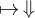Algebraic General Topology. Vol 1: Paperback / E-book || Axiomatic Theory of Formulas: Paperback / E-book

As an Amazon Associate I earn from qualifying purchases.

Point free funcoids as a generalization of frames
by Victor Porton
Email: porton@narod.ru
Web: http://www.mathematics21.org
August 29, 2013
Abstract
I dene an injection from the set of frames to the set of pointfree endo-funcoids.
1 Preliminaries
Read my book  before this article. The preprint of  is not ﬁnal, s o theorem numbers may
change.
2 Confession of a non-professiona l
I am not a professional mathematician. I just recently started my study of pointfree topology by
the book “Stone Spaces”.
I don’t understand how to prove the statement I use below that every frame can be embedded
into a boolean lattice. (I take it below as granted, without under standing the proof.)
Despite of all that, this article presents a new branch of mathematics discovered by me: rela-
tionships between fram e s and locales on one side and pointfree funcoids (p ointfree funcoids are
also my discovery) on an other side. (Frames and locales can be cons idered as a spe cial kind of
pointfree funcoids.) I expect that analyzing pointfree funcoids will turn to be much more easy that
customary ways of doing pointfree topology.
3 Deﬁnitions
3.1 Pointfree funcoid induced by a co-frame
Let L is a co-frame.
We will deﬁne pointfree funcoid L.
Let B(L) is a boolean lattice whose co-subframe L is. (That this mapping exis ts follows from ,
page 53.) There may be probably more than one such mapping, but we just choose one B arbitrarily.
Deﬁne cl(A) =
d
{X L | X A}.
Here
d
can be taken on either L or B(L) as they are the same.
Obvious 1. cl L
B(L)
.
cl(A B) =
d
{X L | X A B} =
d
{X L | X A, X B } =
d
{X
1
X
2
| X
1
A,
X
2
B } =
d
{X
1
| X
1
A}
d
{X
2
| X
2
B} = cl A cl B.
cl 0 = 0 is obvious.
Hence we are under conditions of the theo rem 14.26 in my book.
So there exists a unique pointfree endo-funcoid L FCD(F(B(L)); F(B(L))) such that
h⇑LiX =
l
F(B(L))
hcliup
(F(B(L));P(B(L)))
X
for every ﬁlter X F(B(L)).
13.2 Co-frame induced by a pointfree funcoid
The co- frame f for some pointfree endo-funcoids f will be deﬁ ned to be the reverse of . See
below for exact meaning of being reverse.
Let restore the co-frame L from the pointfree funcoid L.
Let poset f for every pointfree funcoid f is deﬁned by the formula:
f = {X Z(Ob f) | hf iX = X }.
Remark 2. It seems that is not a mo novalued function from pfFCD to Ob(Frm).
3.3 Isomorphis m of co-frames through pointfree funcoids
Remark 3. P(B(L)) = Z(F(B(L))) (theo rem 4.137 in ).
Theorem 4. L
⇓⇑L (where L ranges all sm all frames) is an order isomorphism.
Proof. Let A
⇓⇑L. The n there exists A B(L) s uch that A
=
B(L)
A.
hf iA
=
B(L)
cl A.
hf iA
= A
that is
B(L)
cl A = A
=
B(L)
A. So cl A = A and thus A L.
Let now A L. Then take A
=
B(L)
A. We have hf iA
= cl A =
B(L)
A = A
. So A
⇓⇑L.
We have proved that it is a bijection.
Because A and A
are related by the equation A
=
B(L)
A it is obvious that this is an order
embedding.
4 Postface
Pointfree func oids are a massive generalization of locales and frames: They don’t only require the
lattice of ﬁlters to be boolean but these can be even not lattices of ﬁlters at all but just arbitrary
posets. I think a new era in pointfree topology s tarts.
Much work is yet needed to relate diﬀerent properties o f frames and locales with corresponding
properties of pointfree funcoids.
Bib liography
 Peter T. Johnstone. Stone Spaces. Cambridge University Press, 1982.
 Victor Porton. Algebraic General Topology. Volume 1. 2013.
2 Section